# Division 1

This year, third graders will build a stronger understanding of division. This guided lesson uses the repeated subtraction strategy as a way of teaching division. The lesson shows how division problems can be solved by repeatedly subtracting the same number (the divisor). Not only does this help students solve division problems, but it also builds a conceptual understanding of division. For more practise, check out the suggested division worksheets.
This lesson includes 5 printable learning activities.

## Sir Whiskers Division StorySir Whiskers is considering a newcomer for his cat crew, but she needs to show off some maths skills to be accepted. In this silly story, kids learn all about division in the process. With birthday cupcakes on the line, kids learn division terms and strategies to solve problems by reading about how the new cat helps Sir Whiskers split up items for his crew and the hamster gang.

## Division Facts to 100 with One-Digit Divisors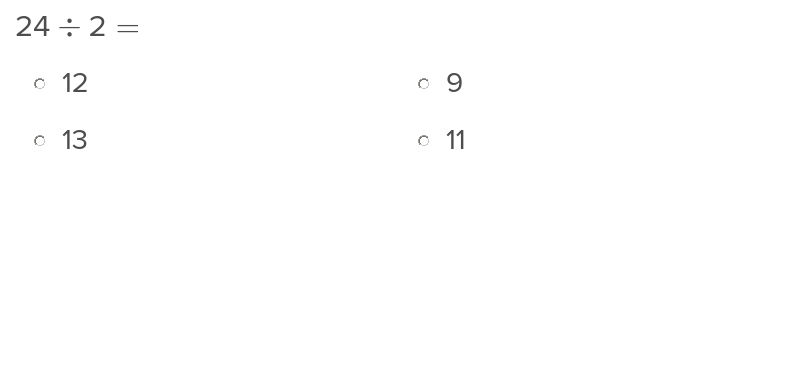Use this exercise to share with students several facts about one digit divisors into numbers up to 100.

## 7s and 8s Division Facts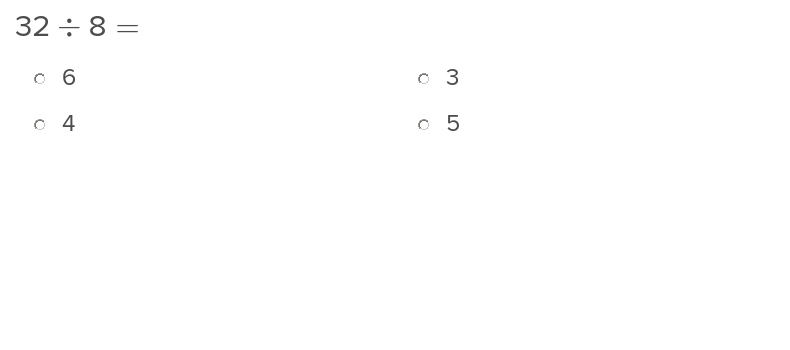Memorizing these one digit divisor facts about 7s and 8s will speed up students’ work on maths problems.

## 9s and 11s Division Facts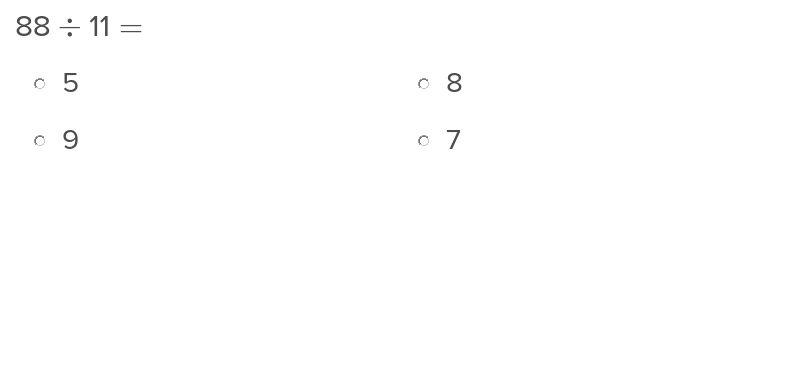After students learn these one digit divisor facts about 9s and 11s, they’ll be able to perform maths problems faster and more accurately.

## Division with One-Digit Divisors and Missing Factors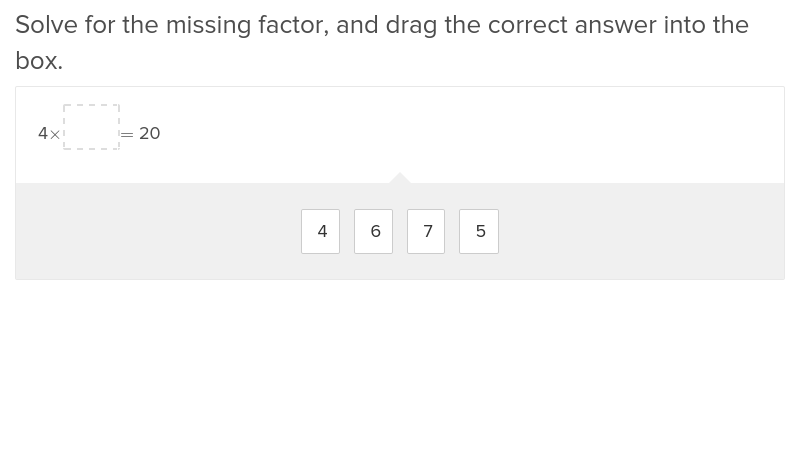Division with One-Digit Divisors and Missing Factors will help students practise this key year four skill.

## 6s and 12s Division Facts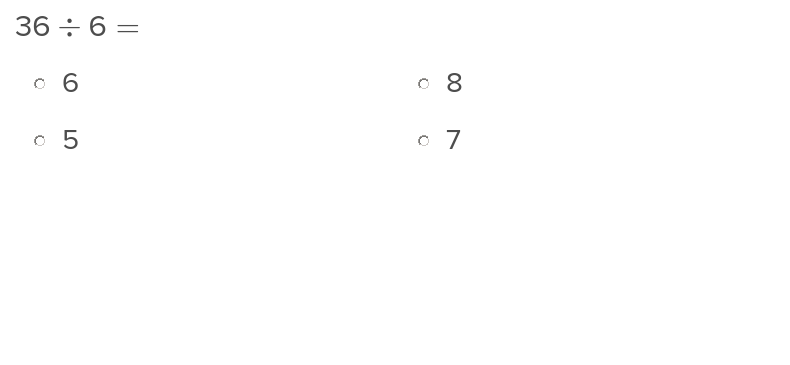Students will be able to simplify maths problems after they learn these division facts about 6s and 12s.

## Division Word Problems with One-Digit Divisors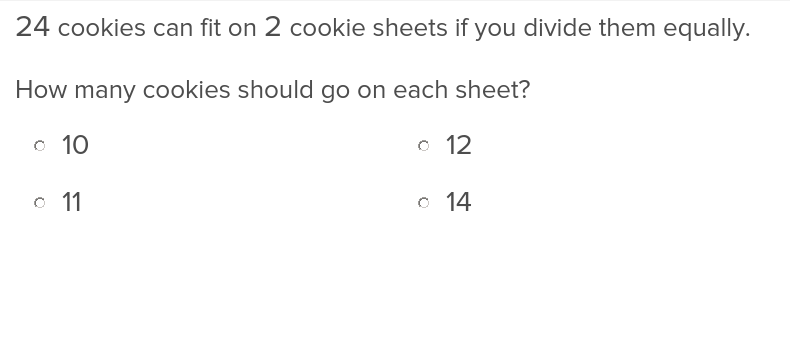Students who work through this exercise will be able to complete division word problems that involve one digit divisors.

Create new collection

0

### New Collection>

0Items

What could we do to improve Education.com?